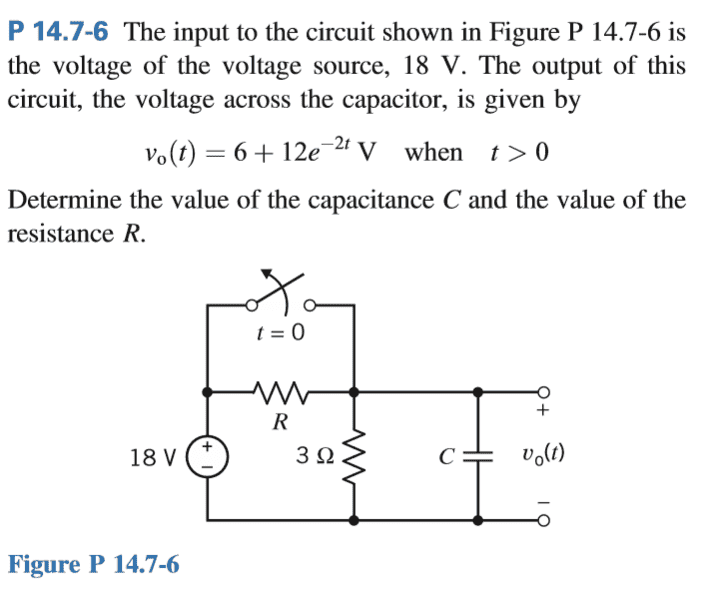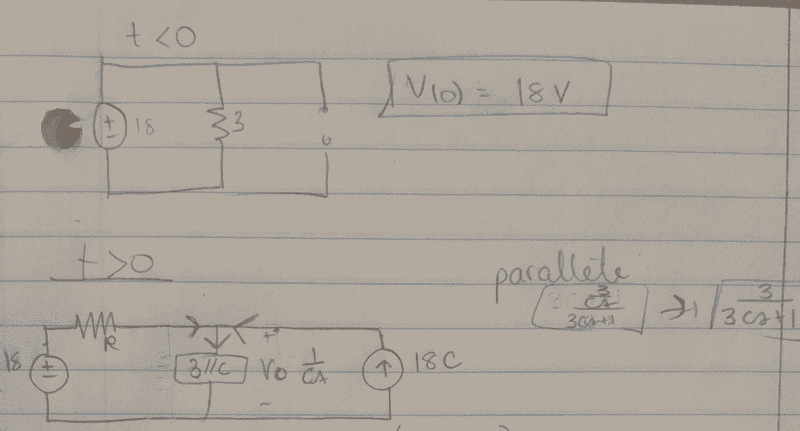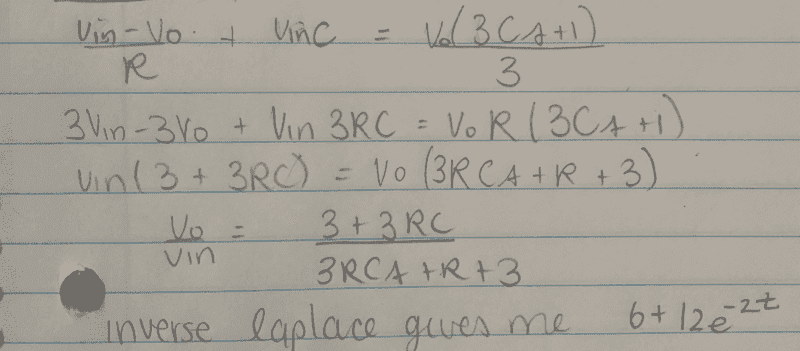# Laplace transforms in circuit analysis, finding missing values

Cocoleia

## Homework Statement## The Attempt at a SolutionAt this point, usually I would replace the values and sometimes separate into partial fractions, and then take the inverse Laplace transformation. So I know that the inverse Laplace needs to give me 6+12e^-2t.

I am given the answers in my notes, R=6ohm and C=0.25F

Is my logic correct, that's to say finding the transfer function and then going about taking an inverse Laplace and kind of "matching" my equation with the unknowns to the equation given to me ?
Thanks

Mentor
Is my logic correct, that's to say finding the transfer function and then going about taking an inverse Laplace and kind of "matching" my equation with the unknowns to the equation given to me ?
I suppose you could go that route. It may be more effort than is warranted though.

Since you're given the time domain response equation you should be able to determine the steady-state (final) voltage. That should let you determine the value of R quite easily. Can you think of what other information the equation gives you that would lead to a value for C?

Cocoleia
I suppose you could go that route. It may be more effort than is warranted though.

Since you're given the time domain response equation you should be able to determine the steady-state (final) voltage. That should let you determine the value of R quite easily. Can you think of what other information the equation gives you that would lead to a value for C?
Is it that I take the Laplace of the given equation:
V(s) = 6/s + 12/(s-2)
and that's how I can say R=6?

Mentor
No Laplace required! Just take the given time domain expression and determine the steady state (final) voltage. Then you should be able to set the value of R in the circuit accordingly. So what is the final voltage?

Hints:
At steady state, what does a capacitor "look like" to the circuit?

What type of circuit remains? (It's a common enough configuration)

Cocoleia
No Laplace required! Just take the given time domain expression and determine the steady state (final) voltage. Then you should be able to set the value of R in the circuit accordingly. So what is the final voltage?

Hints:
At steady state, what does a capacitor "look like" to the circuit?

What type of circuit remains? (It's a common enough configuration)
Do I open circuit the capacitor and then do a voltage divider and since 6 is the "real part" of the given vo, when I solve for R in my voltage divider it equals 6. Solving for R will give 6.

Mentor
Do I open circuit the capacitor and then do a voltage divider and since 6 is the "real part" of the given vo, when I solve for R in my voltage divider it equals 6. Solving for R will give 6.
You should be confident in how to find the steady state conditions of a RC or LC circuit. Yes, you open circuit capacitors and short circuit inductors.

I don't understand what you mean by "real part" of the given vo. The given time domain expression is purely real valued for all time. What's the procedure for finding the steady state value of a time-domain expression? (what does "steady state" imply?)